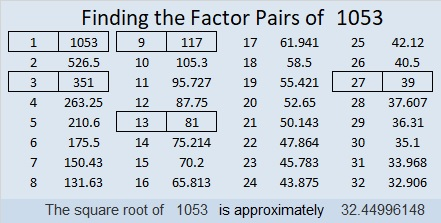# 1053 Find the Factors Challenge

If you can multiply and divide and THINK, then you can solve this puzzle. Go ahead. Give it a try! To solve it, write the numbers from 1 to 10 in each of the four bold areas on the puzzle so that the given clues will be the products of those corresponding factors. Good luck!Print the puzzles or type the solution in this excel file: 12 factors 1044-1053

What can I tell you about the number 1053?

• 1053 is a composite number.
• Prime factorization: 1053 = 3 × 3 × 3 × 3 × 13, which can be written 1053 = 3⁴ × 13
• The exponents in the prime factorization are 4 and 1. Adding one to each and multiplying we get (4 + 1)(1 + 1) = 5 × 2 = 10. Therefore 1053 has exactly 10 factors.
• Factors of 1053: 1, 3, 9, 13, 27, 39, 81, 117, 351, 1053
• Factor pairs: 1053 = 1 × 1053, 3 × 351, 9 × 117, 13 × 81, or 27 × 39
• Taking the factor pair with the largest square number factor, we get √1053 = (√81)(√13) = 9√13 ≈ 32.4499627² + 18²  = 1053 so 1053 is the hypotenuse of a Pythagorean triple:
405-972-1053 calculated from 27² – 18², 2(27)(18), 27² + 18²

1053 is the sum of three consecutive powers of 3:
3 + 3 + 3 = 1053

1053 is 3033 in BASE 7 because 3(7³ + 7¹ + 7º) = 3(351) = 1053, and
it’s palindrome 878 in BASE 11 because 8(121) + 7(11) + 8(1) = 1053